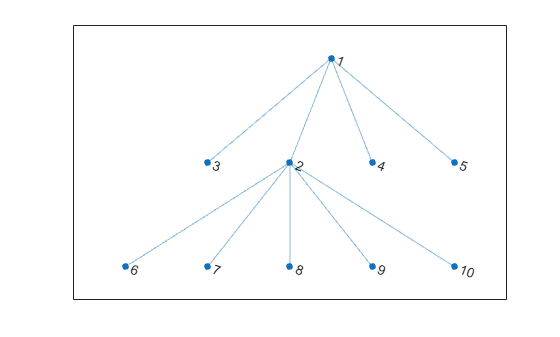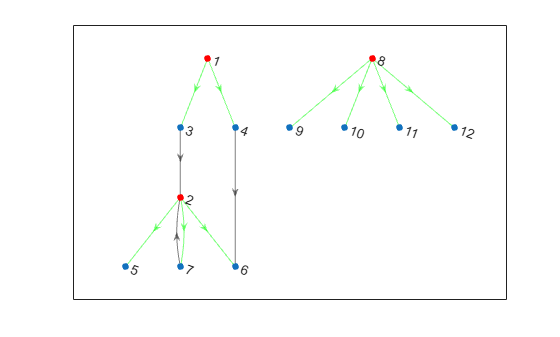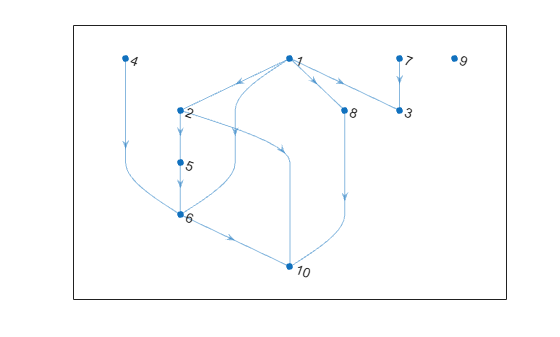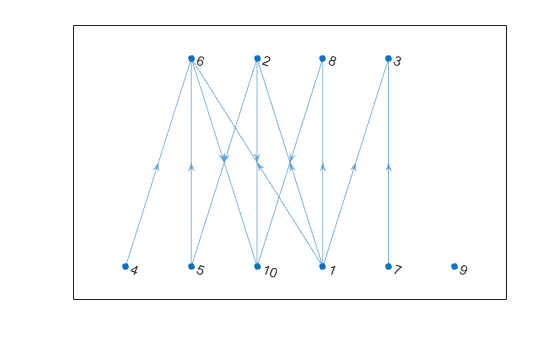# bfsearch

## Syntax

``v = bfsearch(G,s)``
``T = bfsearch(G,s,events)``
``[T,E] = bfsearch(G,s,events)``
``[___] = bfsearch(___,'Restart',tf)``

## Description

example

````v = bfsearch(G,s)` applies breadth-first search to graph `G` starting at node `s`. The result is a vector of node IDs in order of their discovery.```

example

````T = bfsearch(G,s,events)` customizes the output of the breadth-first search by flagging one or more search events. For example, `T = bfsearch(G,s,'allevents')` returns a table containing all flagged events, and `X = bfsearch(G,s,'edgetonew')` returns a matrix or cell array of edges.```
````[T,E] = bfsearch(G,s,events)` additionally returns a vector of edge indices `E` when `events` is set to `'edgetonew'`, `'edgetodiscovered'`, or `'edgetofinished'`. The edge indices are for unique identification of edges in a multigraph.```

example

````[___] = bfsearch(___,'Restart',tf)`, where `tf` is `true`, restarts the search if no new nodes are reachable from the discovered nodes. You can use any of the input or output argument combinations in previous syntaxes. This option ensures that the breadth-first search reaches all nodes and edges in the graph, even if they are not reachable from the starting node, `s`.```

## Examples

collapse all

Create and plot a graph.

```s = [1 1 1 1 2 2 2 2 2]; t = [3 5 4 2 6 10 7 9 8]; G = graph(s,t); plot(G)```Perform a breadth-first search of the graph starting at node 2. The result indicates the order of node discovery.

`v = bfsearch(G,2)`
```v = 10×1 2 1 6 7 8 9 10 3 4 5 ```

Create and plot a directed graph.

```s = [1 1 1 2 3 3 3 4 6]; t = [2 4 5 5 6 7 4 1 4]; G = digraph(s,t); plot(G)```Perform a breadth-first search on the graph starting at node 1. Specify `'allevents'` to return a table containing all of the events in the algorithm.

`T = bfsearch(G,1,'allevents')`
```T=14×4 table Event Node Edge EdgeIndex ________________ ____ __________ _________ startnode 1 NaN NaN NaN discovernode 1 NaN NaN NaN edgetonew NaN 1 2 1 discovernode 2 NaN NaN NaN edgetonew NaN 1 4 2 discovernode 4 NaN NaN NaN edgetonew NaN 1 5 3 discovernode 5 NaN NaN NaN finishnode 1 NaN NaN NaN edgetodiscovered NaN 2 5 4 finishnode 2 NaN NaN NaN edgetofinished NaN 4 1 8 finishnode 4 NaN NaN NaN finishnode 5 NaN NaN NaN ```

To follow the steps in the algorithm, read the events in the table from top to bottom. For example:

1. The algorithm begins at node 1

2. An edge is discovered between node 1 and node 2

3. Node 2 is discovered

4. and so on...

Perform a breadth-first search of a graph with multiple components, and then highlight the graph nodes and edges based on the search results.

Create and plot a directed graph. This graph has two weakly connected components.

```s = [1 1 2 2 2 3 4 7 8 8 8 8]; t = [3 4 7 5 6 2 6 2 9 10 11 12]; G = digraph(s,t); p = plot(G,'Layout','layered');````c = conncomp(G,'Type','weak')`
```c = 1×12 1 1 1 1 1 1 1 2 2 2 2 2 ```

Perform a breadth-first search of the graph starting at node 2, and flag the `'edgetonew'`, `'edgetofinished'`, and `'startnode'` events. Specify `Restart` as `true` to make the search restart whenever there are remaining nodes that cannot be reached.

```events = {'edgetonew','edgetofinished','startnode'}; T = bfsearch(G,2,events,'Restart',true)```
```T=15×4 table Event Node Edge EdgeIndex ______________ ____ __________ _________ startnode 2 NaN NaN NaN edgetonew NaN 2 5 3 edgetonew NaN 2 6 4 edgetonew NaN 2 7 5 edgetofinished NaN 7 2 8 startnode 1 NaN NaN NaN edgetonew NaN 1 3 1 edgetonew NaN 1 4 2 edgetofinished NaN 3 2 6 edgetofinished NaN 4 6 7 startnode 8 NaN NaN NaN edgetonew NaN 8 9 9 edgetonew NaN 8 10 10 edgetonew NaN 8 11 11 edgetonew NaN 8 12 12 ```

When `Restart` is `true`, the `'startnode'` event returns information about where and when the algorithm restarts the search.

Highlight the graph based on event:

• Color the starting nodes red.

• Green edges are for `'edgetonew'`

• Black edges are for `'edgetofinished'`

```highlight(p, 'Edges', T.EdgeIndex(T.Event == 'edgetonew'), 'EdgeColor', 'g') highlight(p, 'Edges', T.EdgeIndex(T.Event == 'edgetofinished'), 'EdgeColor', 'k') highlight(p,T.Node(~isnan(T.Node)),'NodeColor','r')```Use breadth-first search to determine that a graph is bipartite, and return the relevant partitions. A bipartite graph is a graph that has nodes you can divide into two sets, `A` and `B`, with each edge in the graph connecting a node in `A` to a node in `B`.

Create and plot a directed graph.

```s = [1 1 1 1 2 2 4 5 6 7 8]; t = [2 3 6 8 5 10 6 6 10 3 10]; g = digraph(s,t); plot(g);```Use a breadth-first search on the graph to determine if it is bipartite, and if so, return the relevant partitions.

```events = {'edgetonew', 'edgetodiscovered', 'edgetofinished'}; T = bfsearch(g, 1, events, 'Restart', true); partitions = false(1, numnodes(g)); is_bipart = true; is_edgetonew = T.Event == 'edgetonew'; ed = T.Edge; for ii=1:size(T, 1) if is_edgetonew(ii) partitions(ed(ii, 2)) = ~partitions(ed(ii, 1)); else if partitions(ed(ii, 1)) == partitions(ed(ii, 2)) is_bipart = false; break; end end end ```
`is_bipart`
```is_bipart = logical 1 ```

Since `g` is bipartite, the `partitions` variable contains the information about which partition each node belongs to.

Plot the bipartite graph with the `'layered'` layout, using the `partitions` variable to specify the source nodes that appear in the first layer.

`partitions`
```partitions = 1x10 logical array 0 1 1 0 0 1 0 1 0 0 ```
`plot(g, 'Layout', 'layered', 'Source', find(partitions));`## Input Arguments

collapse all

Input graph, specified as either a `graph` or `digraph` object. Use `graph` to create an undirected graph or `digraph` to create a directed graph.

Example: `G = graph(1,2)`

Example: `G = digraph([1 2],[2 3])`

Starting node, specified as one of the values in this table.

ValueExample
Scalar node index`1`
Character vector node name`'A'`
String scalar node name`"A"`

Example: `bfsearch(G,1)`

Flagged search events, specified as one of the options in the following table.

• To flag single events, use the flag names.

• To flag a subset of events, put two or more flag names into a cell array or string array.

• To flag all events, use `'allevents'`.

Note

Depending on the value of `events`, the output of `bfsearch` varies. See the last column in the following table for information about the output returned by each option.

Value of `events`DescriptionOutput
`'discovernode'` (default)

A new node has been discovered.

Return a vector of node IDs:

• If `s` is a numeric node index, then the vector contains numeric node indices.

• If `s` is a node name, then the vector is a cell array containing node names.

`'finishnode'`

All outgoing edges from the node have been visited.

`'startnode'`

This flag indicates the starting node in the search.

If `'Restart'` is `true`, then `'startnode'` flags the starting node each time the search restarts.

`'edgetonew'`

Edge connects to an undiscovered node.

Return a matrix or cell array of size `N`-by-`2` that specifies the end nodes of edges in the graph:

• If `s` is a numeric node index, then the matrix contains numeric node indices.

• If `s` is a node name, then the matrix is a cell array containing node names.

Additionally, you can specify a second output with `[T,E] = bfsearch(…)` that returns a vector of edge indices `E`.

`'edgetodiscovered'`

Edge connects to a previously discovered node.

`'edgetofinished'`

Edge connects to a finished node.

cell array

Specify two or more flags in a cell array to only flag those events during the search.

Return a table, `T`, which contains the variables `T.Event`, `T.Node`, `T.Edge`, and `T.EdgeIndex`:

• `T.Event` is a categorical vector containing the flags in order of their occurrence.

• `T.Node` contains the node ID of the corresponding node for the events `'discovernode'`, `'finishnode'`, and `'startnode'`.

• `T.Edge` contains the corresponding edge for the events `'edgetonew'`, `'edgetodiscovered'`, and `'edgetofinished'`.

• `T.EdgeIndex` contains the edge index for the events `'edgetonew'`, `'edgetodiscovered'`, and `'edgetofinished'`. The edge index is for unique identification of repeated edges in a multigraph.

• Unused elements of `T.Node` and `T.Edge` are set to `NaN`.

• If `s` is a numeric node index, then `T.Node` and `T.Edge` contain numeric node indices.

• If `s` is a node name, then `T.Node` and `T.Edge` are cell arrays containing node names.

`'allevents'`

All events are flagged.

Example: `v = bfsearch(G,3)` begins the search at the third node and returns a vector, `v`, containing the nodes in order of discovery. This is the same as `v = bfsearch(G,3,'discovernode')`.

Example: `X = bfsearch(G,'A','edgetonew')` begins at the node named `'A'` and returns a cell array, `X`, indicating each of the edges that connects to an undiscovered node during the search.

Example: `T = bfsearch(G,s,{'discovernode','finishnode'})` returns a table, `T`, but only flags when new nodes are discovered or when a node is marked finished.

Example: `T = bfsearch(G,s,'allevents')` flags all search events and returns a table, `T`.

Data Types: `char` | `string` | `cell`

Toggle to restart search, specified as `false` (default) or `true`. This option is useful if the graph contains nodes that are unreachable from the starting node. If `'Restart'` is `true`, then the search restarts whenever undiscovered nodes remain that are unreachable from the discovered nodes. The new start node is the node with smallest index that is still undiscovered. The restarting process repeats until `bfsearch` discovers all nodes.

`'Restart'` is `false` by default, so that the search only visits nodes that are reachable from the starting node.

When `'Restart'` is `true`, the `'discovernode'` and `'finishnode'` events occur once for each node in the graph. Also, each edge in the graph is flagged once by `'edgetonew'`, `'edgetodiscovered'`, or `'edgetofinished'`. The edges flagged by `'edgetonew'` form one or more trees.

Example: `T = bfsearch(graph([1 3],[2 4]),1,'Restart',true)` searches both of the connected components in the graph.

Data Types: `logical`

## Output Arguments

collapse all

Node IDs, returned in one of the following formats:

• If you use a numeric node ID to specify the starting node `s`, then `v` is a numeric column vector of node indices.

• If `s` is a character vector or string containing a node name, then `v` is a cell vector containing node names.

The node IDs in `v` reflect the order of discovery by the breadth-first graph search.

Search results, returned in one of the following formats:

• If `events` is not specified or is `'discovernode'`, `'finishnode'`, or `'startnode'`, then `T` is a vector of node IDs similar to `v`.

• If `events` is `'edgetonew'`, `'edgetodiscovered'`, or `'edgetofinished'`, then `T` is a matrix or cell array of size `N`-by-`2` indicating the source and target nodes for each relevant edge.

• If `events` is a cell array of search events or `'allevents'`, then `T` is a table containing the flagged search events. The table contains the search event flags in `T.Event`, relevant node IDs in `T.Node`, and relevant edges in `T.Edge` and `T.EdgeIndex`.

In all cases:

• The order of the elements or rows of `T` indicates their order of occurrence during the search.

• If you specify `s` as a numeric node ID, then `T` also refers to nodes using their numeric IDs.

• If you specify `s` as a node name, then `T` also refers to nodes using their names.

Edge indices, returned as a vector.

Specify this output to get a vector of edge indices for the events `'edgetonew'`, `'edgetodiscovered'`, or `'edgetofinished'`. The `N`-by-`1` vector of edge indices corresponds with `T`, which is a matrix or cell array of size `N`-by-`2` indicating the source and target nodes for each relevant edge.

Example: `[T,E] = bfsearch(G,s,'edgetonew')`

## Tips

• `dfsearch` and `bfsearch` treat undirected graphs the same as directed graphs. An undirected edge between nodes `s` and `t` is treated like two directed edges, one from `s` to `t` and one from `t` to `s`.

## Algorithms

The Breadth-First search algorithm begins at the starting node, `s`, and inspects all of its neighboring nodes in order of their node index. Then for each of those neighbors, it visits their unvisited neighbors in order. The algorithm continues until all nodes that are reachable from the starting node have been visited.

In pseudo-code, the algorithm can be written as:

```Event startnode(S) Event discovernode(S) NodeList = {S} WHILE NodeList is not empty C = NodeList{1} Remove first element from NodeList FOR edge E from outgoing edges of node C, connecting to node N Event edgetonew(C,E), edgetodiscovered(C,E) or edgetofinished(C,E) (depending on the state of node N) IF event was edgetonew Event discovernode(N) Append N to the end of NodeList END END Event finishnode(C) END ```

`bfsearch` can return flags to describe the different events in the algorithm, such as when a new node is discovered or when all of the outgoing edges of a node have been visited. The event flags are listed here.

Event FlagEvent Description
`'discovernode'`

A new node has been discovered.

`'finishnode'`

All outgoing edges from the node have been visited.

`'startnode'`

This flag indicates the starting node in the search.

`'edgetonew'`

Edge connects to an undiscovered node

`'edgetodiscovered'`

Edge connects to a previously discovered node

`'edgetofinished'`

Edge connects to a finished node

For more information, see the input argument description for `events`.

Note

In cases where the input graph contains nodes that are unreachable from the starting node, the `'Restart'` option provides a way to make the search visit every node in the graph. In that case, the `'startnode'` event indicates the starting node each time the search restarts.

## Version History

Introduced in R2015b